# Lecture 1 C Basics I Variable Classes Seoul

• Slides: 24
Download presentationLecture 1 C++ Basics I Variable Classes Seoul National University Graphics & Media Lab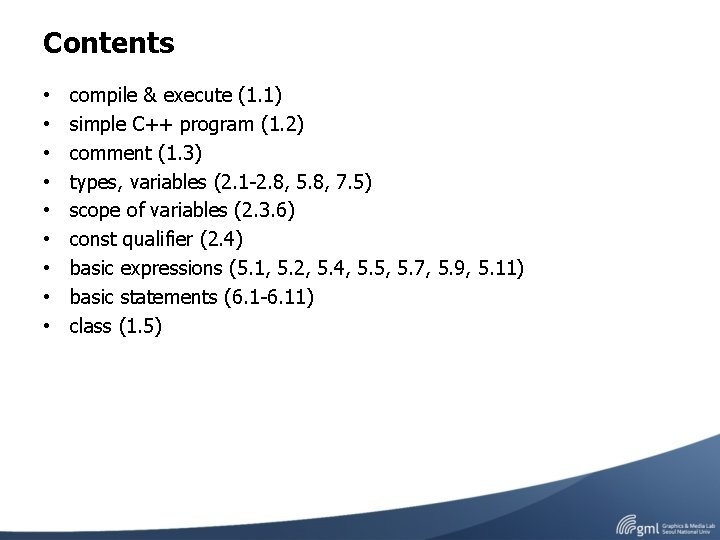Contents • • • compile & execute (1. 1) simple C++ program (1. 2) comment (1. 3) types, variables (2. 1 -2. 8, 5. 8, 7. 5) scope of variables (2. 3. 6) const qualifier (2. 4) basic expressions (5. 1, 5. 2, 5. 4, 5. 5, 5. 7, 5. 9, 5. 11) basic statements (6. 1 -6. 11) class (1. 5)Compile & Execute Write or edit source code Compile source code Link object code Run program Fix bugs that emerge during compilation Fix bugs that emerge during execution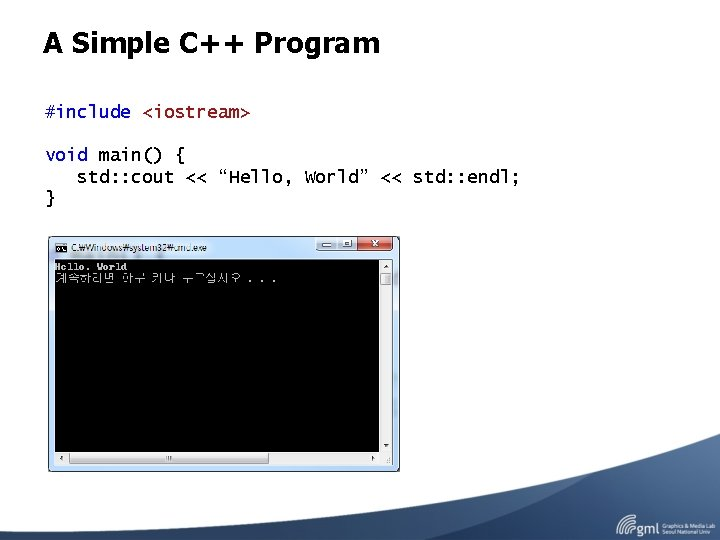A Simple C++ Program #include <iostream> void main() { std: : cout << “Hello, World” << std: : endl; }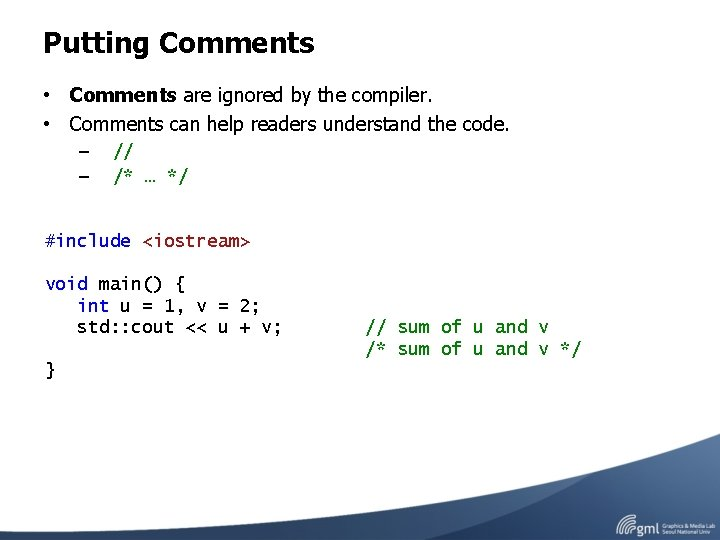Putting Comments • Comments are ignored by the compiler. • Comments can help readers understand the code. – – // /* … */ #include <iostream> void main() { int u = 1, v = 2; std: : cout << u + v; } // sum of u and v /* sum of u and v */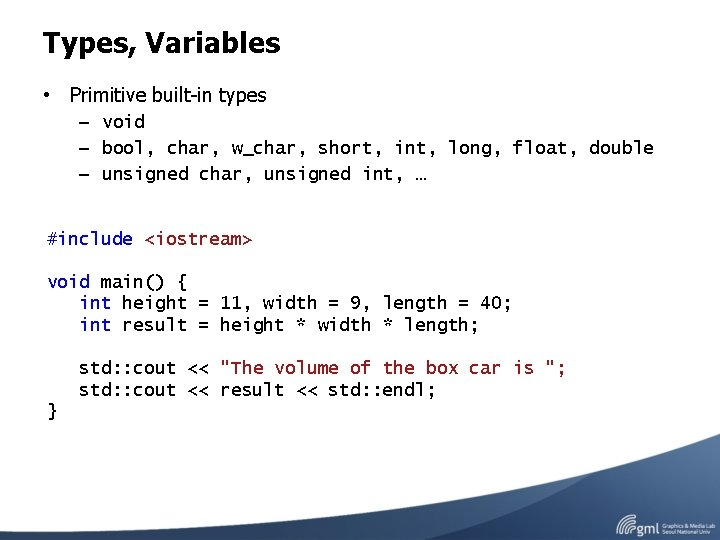Types, Variables • Primitive built-in types – void – bool, char, w_char, short, int, long, float, double – unsigned char, unsigned int, … #include <iostream> void main() { int height = 11, width = 9, length = 40; int result = height * width * length; std: : cout << "The volume of the box car is "; std: : cout << result << std: : endl; }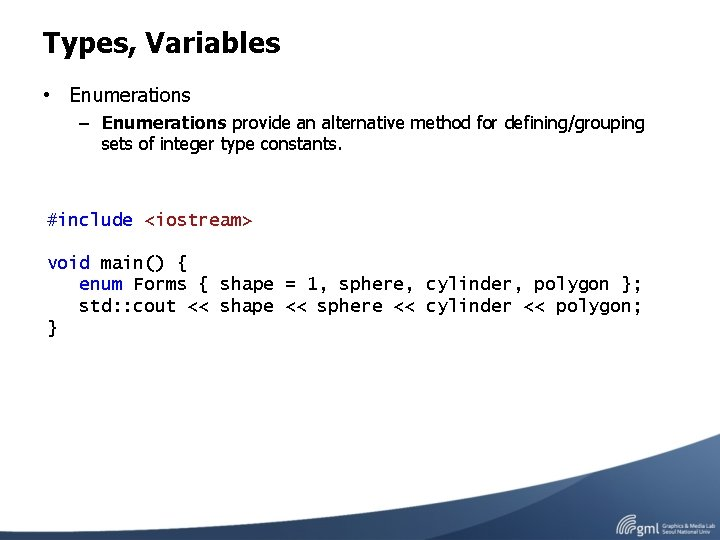Types, Variables • Enumerations – Enumerations provide an alternative method for defining/grouping sets of integer type constants. #include <iostream> void main() { enum Forms { shape = 1, sphere, cylinder, polygon }; std: : cout << shape << sphere << cylinder << polygon; }Types, Variables • typedef – typedef allows us to define a synonym for a type #include <iostream> typedef double wages; typedef int exam_score; typedef wages salary; void main() { wages exam_score // wages is double // exam_score is int // salary is wages (double) wage 0 = 200, wage 1 = 300; score 0 = 90, score 1 = 100; std: : cout << wages 0 << score 0; std: : cout << wages 1 << score 1; }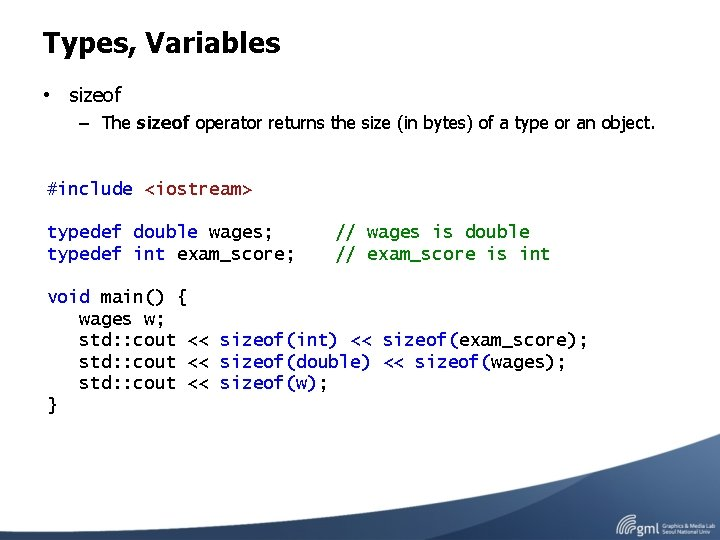Types, Variables • sizeof – The sizeof operator returns the size (in bytes) of a type or an object. #include <iostream> typedef double wages; typedef int exam_score; // wages is double // exam_score is int void main() { wages w; std: : cout << sizeof(int) << sizeof(exam_score); std: : cout << sizeof(double) << sizeof(wages); std: : cout << sizeof(w); }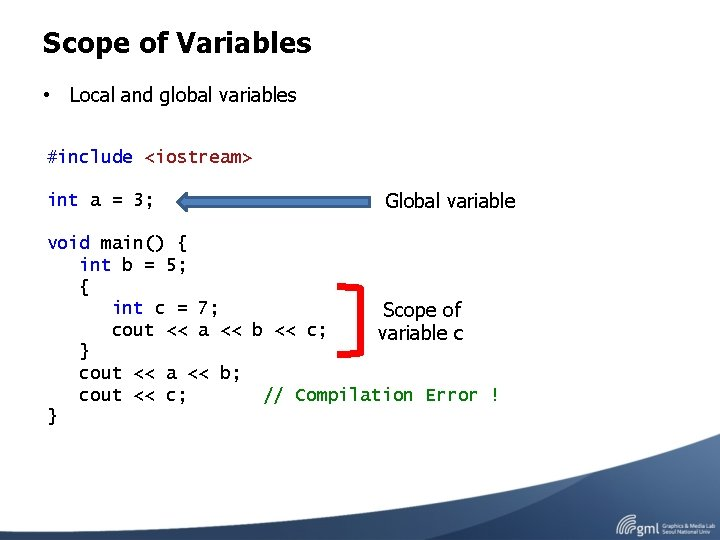Scope of Variables • Local and global variables #include <iostream> int a = 3; Global variable void main() { int b = 5; { int c = 7; Scope of cout << a << b << c; variable c } cout << a << b; cout << c; // Compilation Error ! }Scope of Variables • extern – We can declare a global variable without defining it by using the extern keyword. main. cpp tmp. cpp #include <iostream> int a = 3; extern int a; declaration of a void main() { std: : cout << a << std: : endl; } definition of a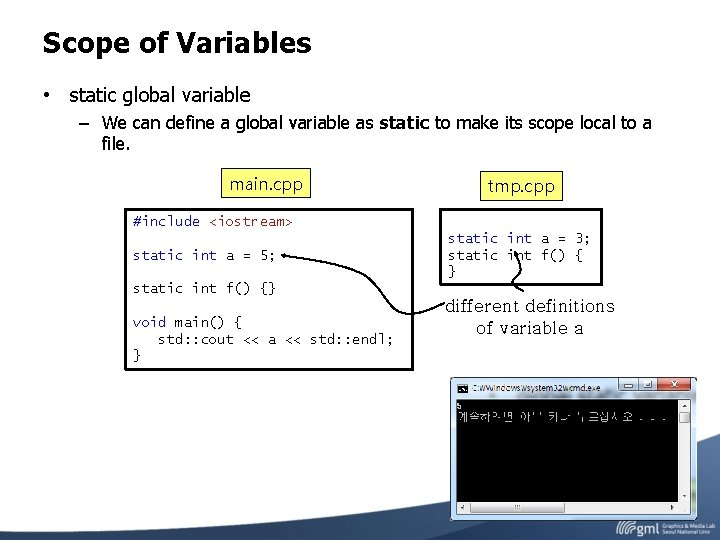Scope of Variables • static global variable – We can define a global variable as static to make its scope local to a file. main. cpp tmp. cpp #include <iostream> static int a = 5; static int a = 3; static int f() { } static int f() {} void main() { std: : cout << a << std: : endl; } different definitions of variable a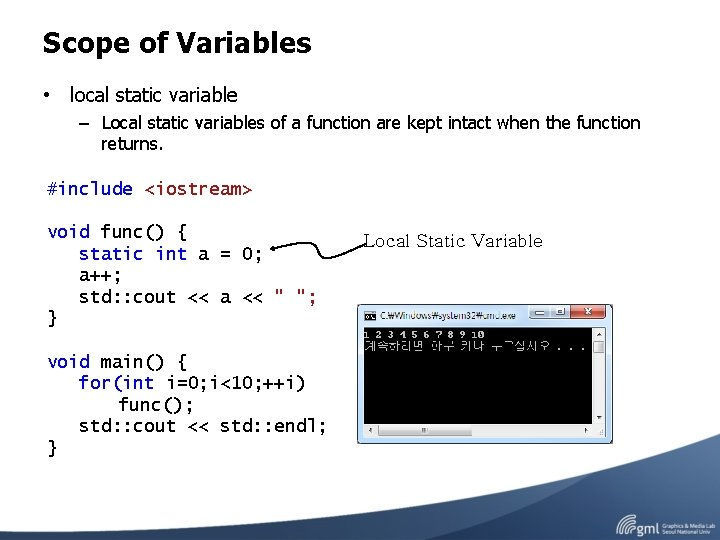Scope of Variables • local static variable – Local static variables of a function are kept intact when the function returns. #include <iostream> void func() { static int a = 0; a++; std: : cout << a << " "; } void main() { for(int i=0; i<10; ++i) func(); std: : cout << std: : endl; } Local Static VariableConst Qualifier • const – A constant is a special kind of variable whose value cannot be altered in the program. #include <iostream> void main() { int a = 3; const int b = 5; a = 7; b = 7; } // b is const variable // Compilation Error !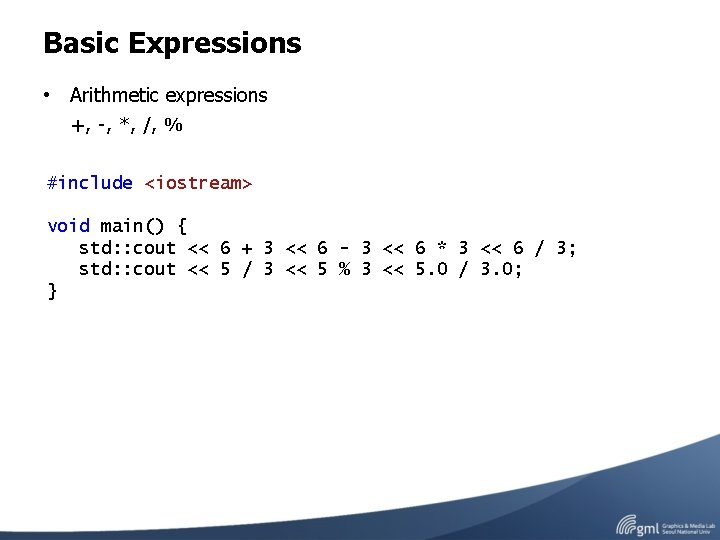Basic Expressions • Arithmetic expressions +, -, *, /, % #include <iostream> void main() { std: : cout << 6 + 3 << 6 - 3 << 6 * 3 << 6 / 3; std: : cout << 5 / 3 << 5 % 3 << 5. 0 / 3. 0; }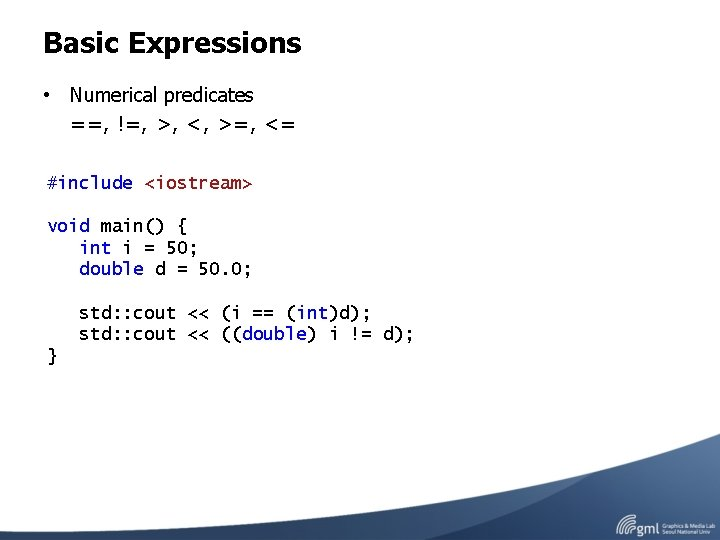Basic Expressions • Numerical predicates ==, !=, >, <, >=, <= #include <iostream> void main() { int i = 50; double d = 50. 0; std: : cout << (i == (int)d); std: : cout << ((double) i != d); }Basic Expressions • Conditional operator cond ? expr 1 : expr 2; #include <iostream> void main() { int score; std: : cin >> score; std: : cout << “The score is “ << score << (score==1 ? “ point” : “ points”) << “. ” << std: : endl; }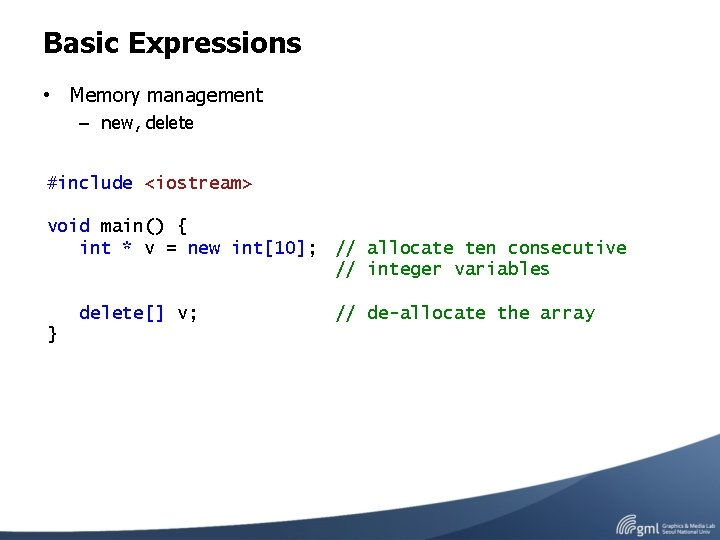Basic Expressions • Memory management – new, delete #include <iostream> void main() { int * v = new int; // allocate ten consecutive // integer variables delete[] v; } // de-allocate the array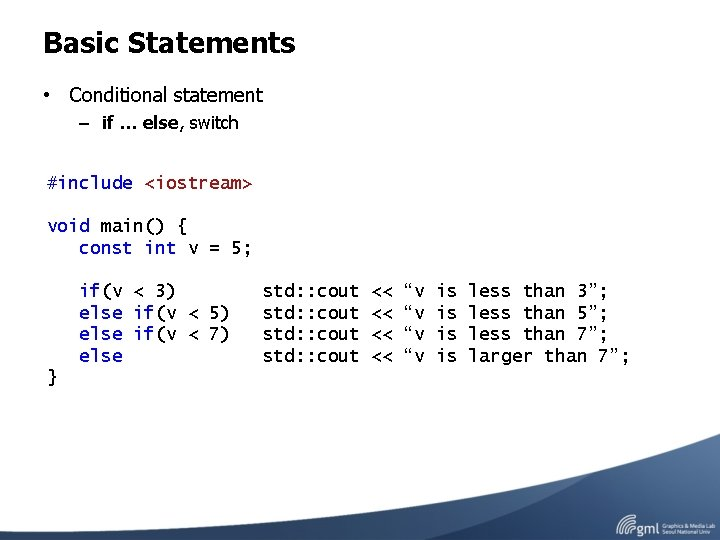Basic Statements • Conditional statement – if … else, switch #include <iostream> void main() { const int v = 5; if(v < 3) else if(v < 5) else if(v < 7) else } std: : cout << << “v “v is is less than 3”; less than 5”; less than 7”; larger than 7”;Basic Statements • Conditional statement – if … else, switch #include <iostream> void main() { const int v = 5; switch(v) { case 3: case 5: case 7: default : } } std: : cout << << “v “v is is 3”; 5”; 7”; not break; 3 or 5 or 7”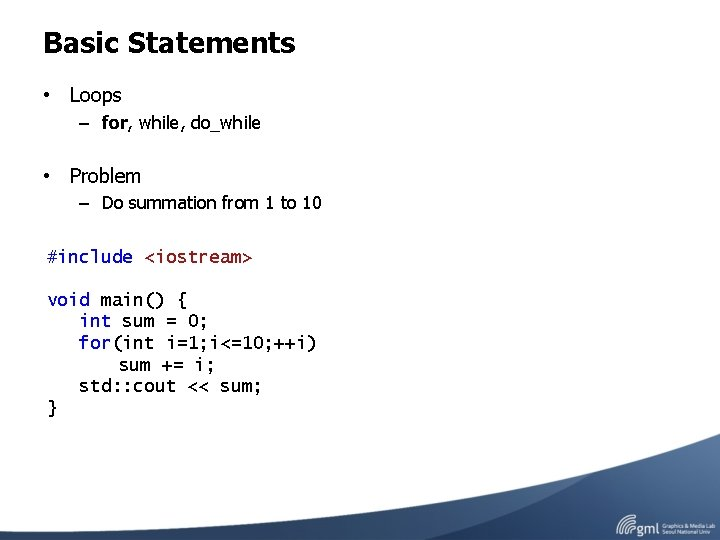Basic Statements • Loops – for, while, do_while • Problem – Do summation from 1 to 10 #include <iostream> void main() { int sum = 0; for(int i=1; i<=10; ++i) sum += i; std: : cout << sum; }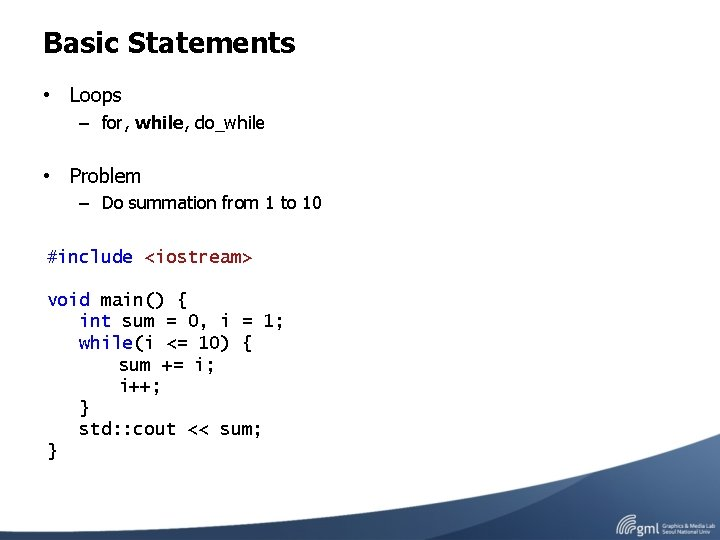Basic Statements • Loops – for, while, do_while • Problem – Do summation from 1 to 10 #include <iostream> void main() { int sum = 0, i = 1; while(i <= 10) { sum += i; i++; } std: : cout << sum; }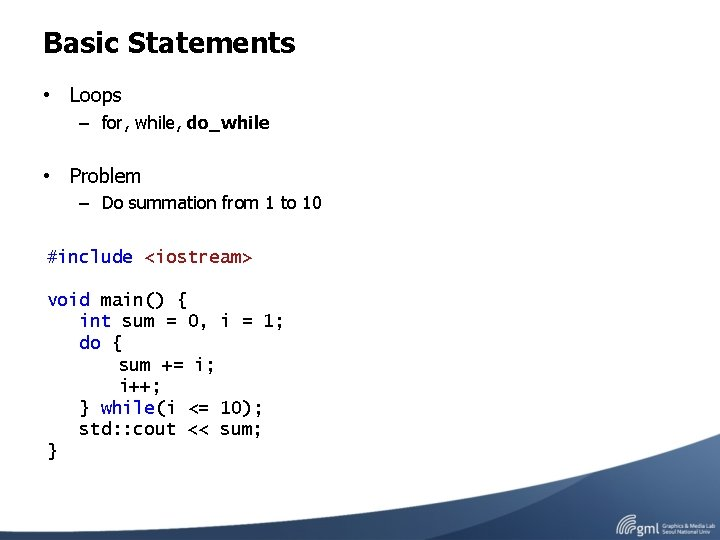Basic Statements • Loops – for, while, do_while • Problem – Do summation from 1 to 10 #include <iostream> void main() { int sum = 0, i = 1; do { sum += i; i++; } while(i <= 10); std: : cout << sum; }Class • A class consists of the datafields and interface. #include <iostream> class Box { public: void print() { std: : cout << height << " " << width << " " << length << std: : endl; } double height, width, length; }; void main() { Box box; box. height = 3; box. width = 5; box. length = 7; box. print(); }